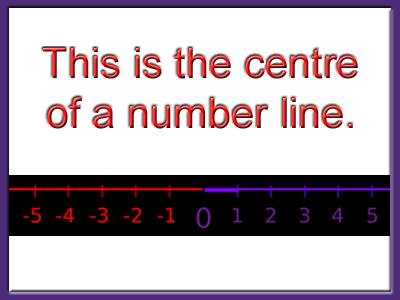Number Lines and Solution Sets

This Math quiz is called 'Number Lines and Solution Sets' and it has been written by teachers to help you if you are studying the subject at middle school. Playing educational quizzes is a fabulous way to learn if you are in the 6th, 7th or 8th grade - aged 11 to 14.

It costs only \$12.50 per month to play this quiz and over 3,500 others that help you with your school work. You can subscribe on the page at Join Us

Number lines are a great aid to tricky calculations, particularly if they involve negative numbers. Traditionally, our number lines are horizontal, with negative numbers to the left of zero, and positive numbers to the right.

You will also be familiar with vertical number lines, in thermometer scales and height/depth levels. Number lines are useful to help illustrate the solution to an inequality. See how well you can do in this middle school Math quiz!

If you have tried the Linear Inequalities quiz you will know that an inequality has a range of solutions. This can be displayed on a number line, with a circle drawn at the number that is the limit, and an arrow to indicate all numbers above or below the limit. Whether or not the circle is filled in or left open will depend on the nature of the inequality.

If the solution is ‘greater than’ (>) or ‘less than’ (<) then the circle stays open. If the solution includes ‘equal’, as in ≤ (less than or equal to) and ≥ (greater than or equal to) then the circle should be filled in. The direction of the arrow is important – ‘Less than’ goes Left, ‘gReater than’ goes Right.

When an inequality is bounded by an upper and a lower limit, such as -2 < x ≤ 5, this can be drawn as a line with a circle at each end – open at -2, and closed at 5.

If you use a number line to help work out a sum involving negative numbers, it doesn’t need to be drawn to scale. In fact, it doesn’t need all the numbers written in, just the key ones – the numbers from the question, plus the all-important zero. Then decide if you are moving left or right from the start point, and do you go across the zero line?

Question 1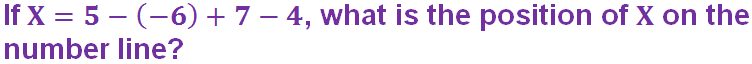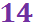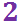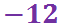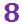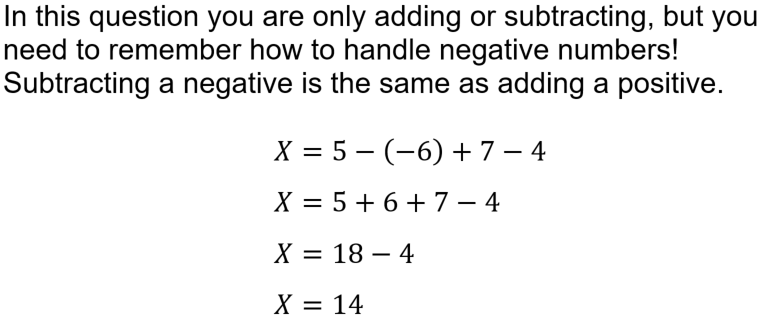Question 2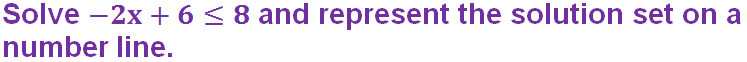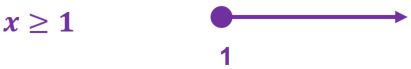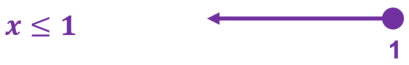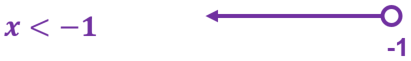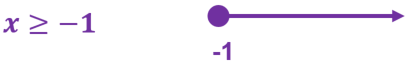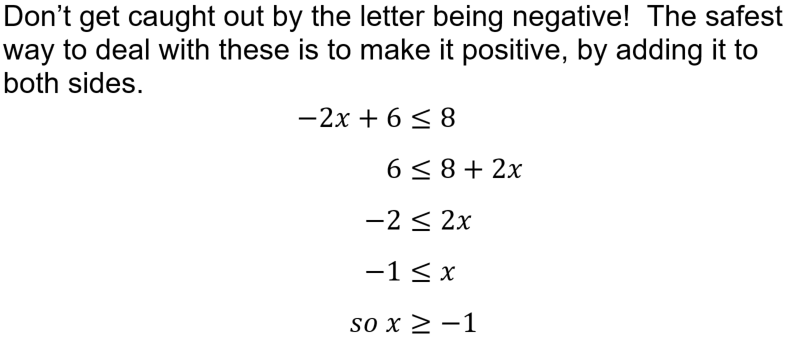Question 3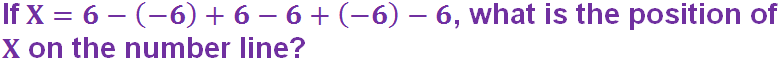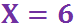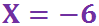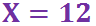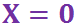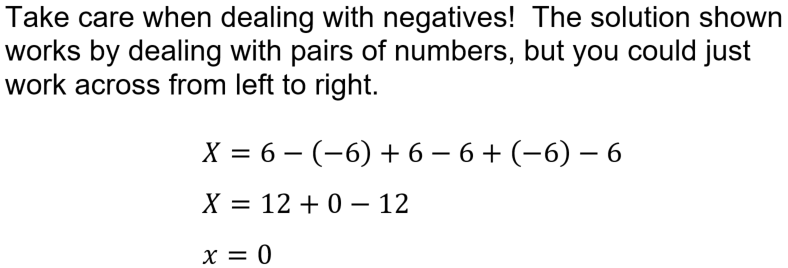Question 4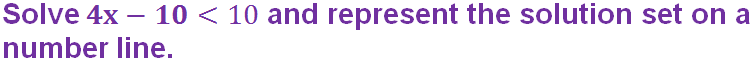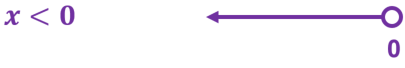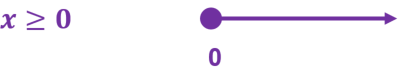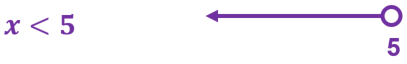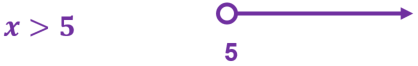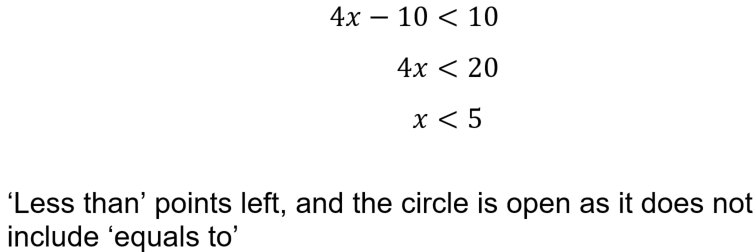Question 5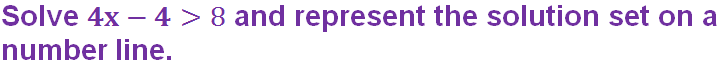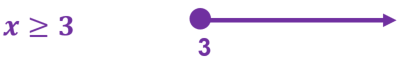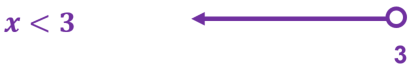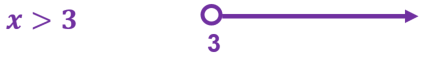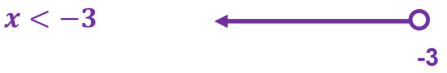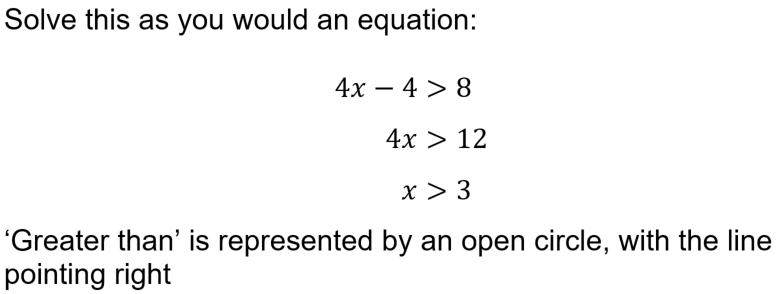Question 6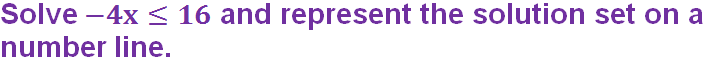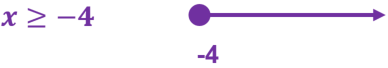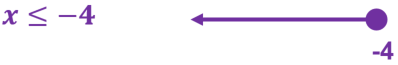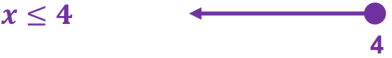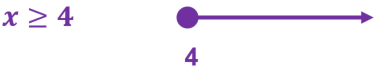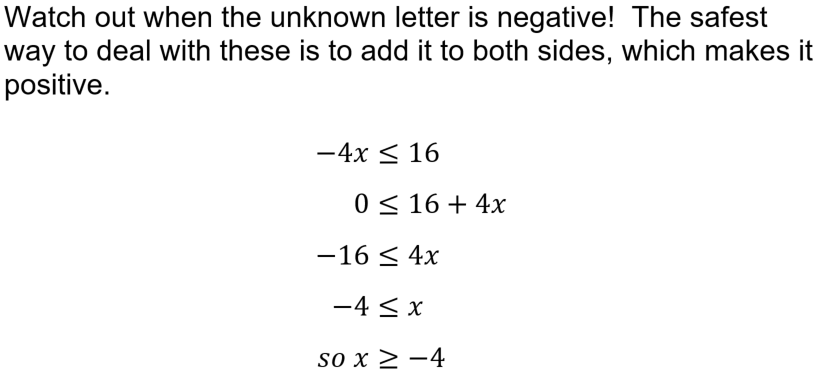Question 7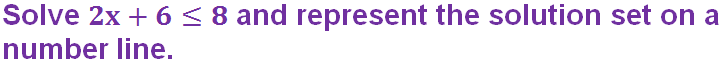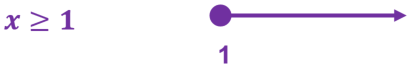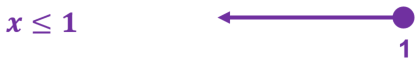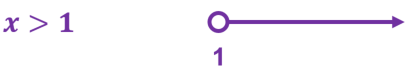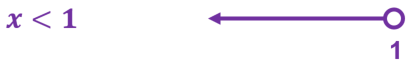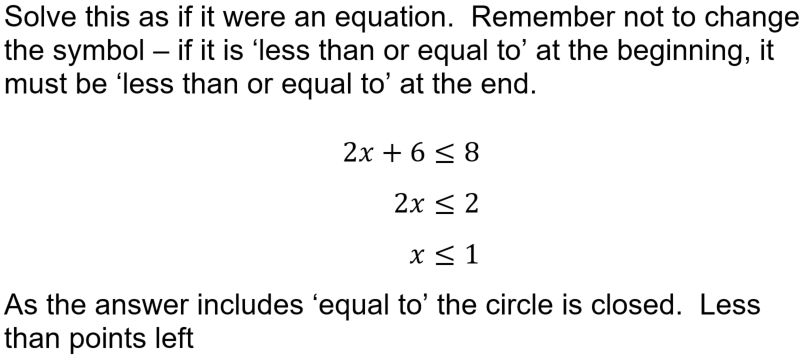Question 8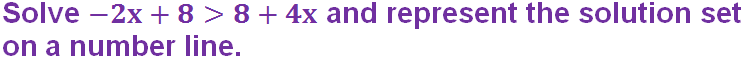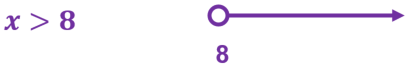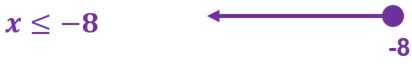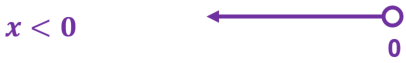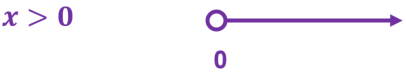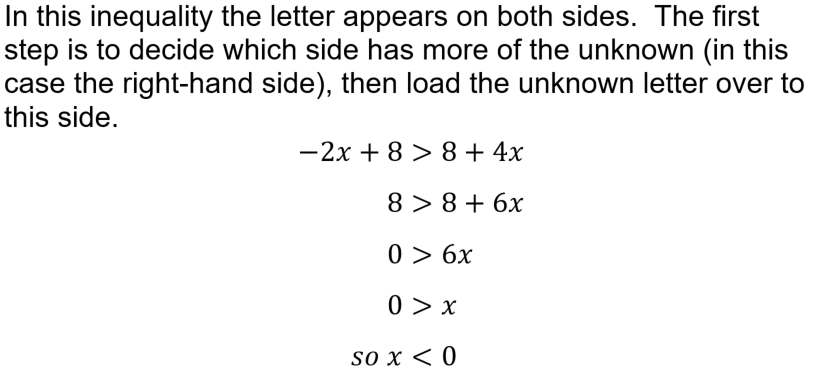Question 9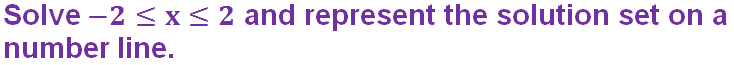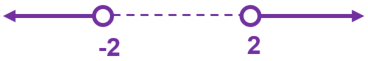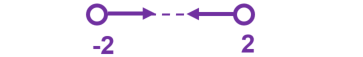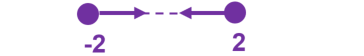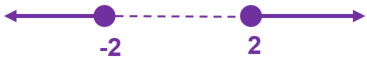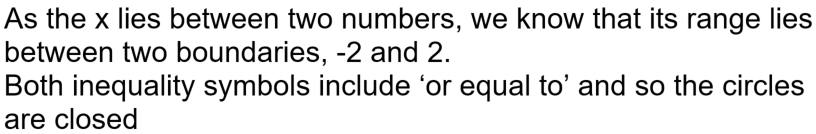Question 10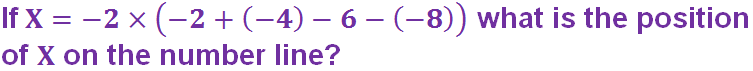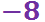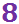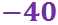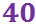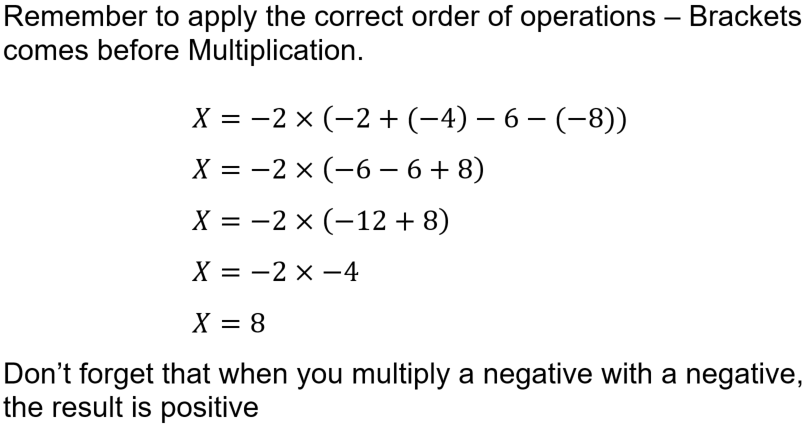Author:  Sally Thompson

We use cookies to make your experience of our website better.

To comply with the new e-Privacy directive, we need to ask for your consent -